# cogeneration module¶

This module is designed to hold functions for pre- and postprocessing for combined heat and power plants.

This file is part of project oemof (github.com/oemof/oemof-thermal). It’s copyrighted by the contributors recorded in the version control history of the file, available from its original location: oemof-thermal/src/oemof/thermal/chp.py

oemof.thermal.cogeneration.allocate_emissions(total_emissions, eta_el, eta_th, method, **kwargs)[source]

Function to allocate emissions caused in cogeneration to the products electrical energy and heat according to specified method.

IEA method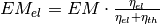,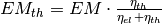.

Efficiency method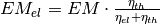,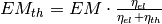.

Finnish method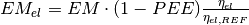,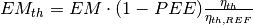,

with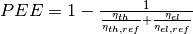.

Reference: Mauch, W., Corradini, R., Wiesmeyer, K., Schwentzek, M. (2010). Allokationsmethoden für spezifische CO2-Emissionen von Strom und Waerme aus KWK-Anlagen. Energiewirtschaftliche Tagesfragen, 55(9), 12–14.

Parameters: total_emissions (numeric) – Total absolute emissions to be allocated to electricity and heat [in CO2 equivalents]. eta_el (numeric) – Electrical efficiency of the cogeneration [-]. eta_th (numeric) – Thermal efficiency of the cogeneration [-]. method (str) – Specification of method to use. Choose from [‘iea’, finnish’, ‘efficiency’]. **kwargs – For the finnish method, eta_el_ref and eta_th_ref have to be passed. allocated_emissions_electricity (numeric) – total emissions allocated to electricity according to specified method [in CO2 equivalents]. allocated_emissions_heat (numeric) – total emissions allocated to heat according to specified method [in CO2 equivalents].

# concentrating_solar_power module¶

This module is designed to hold functions which are necessary for the CSP.

This file is part of project oemof (github.com/oemof/oemof-thermal). It’s copyrighted by the contributors recorded in the version control history of the file, available from its original location: oemof-thermal/src/oemof/thermal/concentrating_solar_power.py

oemof.thermal.concentrating_solar_power.calc_collector_irradiance(irradiance_on_collector, cleanliness)[source]

Subtracts the losses of dirtiness from the irradiance on the collector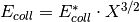Parameters: irradiance_on_collector (series of numeric) – Irradiance which hits collectors surface. x (numeric) – Cleanliness of the collector (between 0 and 1). collector_irradiance – Irradiance on collector after all losses. series of numeric
oemof.thermal.concentrating_solar_power.calc_eta_c(eta_0, c_1, c_2, iam, temp_collector_inlet, temp_collector_outlet, temp_amb, collector_irradiance, loss_method)[source]

Calculates collectors efficiency depending on the loss method

method ‘Janotte’: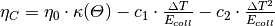method ‘Andasol’: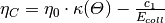Parameters: eta_0 (numeric) – Optical efficiency of the collector. c_1 (numeric) – Thermal loss parameter 1. Required for both loss methods. c_2 (numeric) – Thermal loss parameter 2. Required for loss method ‘Janotte’. iam (series of numeric) – Incidence angle modifier. temp_collector_inlet (numeric, in °C) – Collectors inlet temperature. temp_collector_outlet (numeric, in °C) – Collectors outlet temperature. temp_amb (series of numeric, in °C) – Ambient temperature. collector_irradiance (series of numeric) – Irradiance on collector after all losses. loss_method (string, default 'Janotte') – Valid values are: ‘Janotte’ or ‘Andasol’. Describes, how the thermal losses and the incidence angle modifier are calculated. collectors efficiency series of numeric
oemof.thermal.concentrating_solar_power.calc_heat_coll(eta_c, collector_irradiance)[source]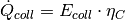Parameters: eta_c (series of numeric) – collectors efficiency. collector_irradiance (series of numeric) – Irradiance on collector after all losses. collectors heat series of numeric
oemof.thermal.concentrating_solar_power.calc_iam(a_1, a_2, a_3, a_4, a_5, a_6, aoi, loss_method)[source]

Calculates the incidence angle modifier depending on the loss method

method ‘Janotte’: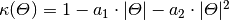method ‘Andasol’: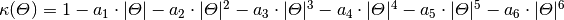Parameters: a_2, a_3, a_4, a_5, a_6 (a_1,) – Parameters for the incident angle modifier. For loss method ‘Janotte’ a_1 and a_2 are required, for ‘Andasol’ a_1 to a_6 are required. aoi (series of numeric) – Angle of incidence. loss_method (string, default 'Janotte') – Valid values are: ‘Janotte’ or ‘Andasol’. Describes, how the thermal losses and the incidence angle modifier are calculated. Incidence angle modifier series of numeric
oemof.thermal.concentrating_solar_power.calc_irradiance(surface_tilt, surface_azimuth, apparent_zenith, azimuth, irradiance, irradiance_method)[source]
Parameters: surface_tilt (series of numeric) – Panel tilt from horizontal. surface_azimuth (series of numeric) – Panel azimuth from north. apparent_zenith (series of numeric) – Solar zenith angle. azimuth (series of numeric) – Solar azimuth angle. irradiance (series of numeric) – Solar irraciance (dni or E_direct_horizontal). irradiance_method (str) – Describes, if the horizontal direct irradiance or the direct normal irradiance is given and used for calculation. irradiance_on_collector – Irradiance which hits collectors surface. series of numeric
oemof.thermal.concentrating_solar_power.csp_precalc(lat, long, collector_tilt, collector_azimuth, cleanliness, eta_0, c_1, c_2, temp_collector_inlet, temp_collector_outlet, temp_amb, a_1, a_2, a_3=0, a_4=0, a_5=0, a_6=0, loss_method='Janotte', irradiance_method='horizontal', **kwargs)[source]

Calculates collectors efficiency and irradiance according to  and the heat of the thermal collector. For the calculation of irradiance pvlib  is used.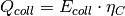functions used
• pvlib.solarposition.get_solarposition
• pvlib.tracking.singleaxis
• calc_iam
• calc_eta_c
• calc_heat_coll
Parameters: lat (numeric) – Latitude of the location. long (numeric) – Longitude of the location. collector_tilt (numeric) – The tilt of the collector. collector_azimuth (numeric) – The azimuth of the collector. Azimuth according to pvlib in decimal degrees East of North. cleanliness (numeric) – Cleanliness of the collector (between 0 and 1). a_2, a_3, a_4, a_5, a_6 (a_1,) – Parameters for the incident angle modifier. For loss method ‘Janotte’ a_1 and a_2 are required, for ‘Andasol’ a_1 to a_6 are required. eta_0 (numeric) – Optical efficiency of the collector. c_1 (numeric) – Thermal loss parameter 1. Required for both loss methods. c_2 (numeric) – Thermal loss parameter 2. Required for loss method ‘Janotte’. If loss method ‘Andasol’ is used, set it to 0. temp_collector_inlet (numeric or series with length periods) – Collectors inlet temperature. temp_collector_outlet (numeric or series with length periods) – Collectors outlet temperature. temp_amb (time indexed series) – Ambient temperature time series. loss_method (string, default 'Janotte') – Valid values are: ‘Janotte’ or ‘Andasol’. Describes, how the thermal losses and the incidence angle modifier are calculated. irradiance_method (string, default 'horizontal') – Valid values are: ‘horizontal’ or ‘normal’. Describes, if the horizontal direct irradiance or the direct normal irradiance is given and used for calculation. (depending on irradiance_method) (E_dir_hor/dni) – Irradiance for calculation. data – Dataframe containing the following columns collector_irradiance eta_c collector_heat collector_irradiance is the irradiance which reaches the collector after all losses (incl. cleanliness). pandas.DataFrame

Comment

Series for ambient temperature and irradiance must have the same length and the same time index. Be aware of the time one.

Proposal of values

If you have no idea, which values your collector have, here are values, which were measured in  for a collector: a1: -0.00159, a2: 0.0000977, eta_0: 0.816, c1: 0.0622, c2: 0.00023.

Reference

 Janotte, N; et al: Dynamic performance evaluation of the HelioTrough collector demon-stration loop - towards a new benchmark in parabolic trough qualification, SolarPACES 2013

 William F. Holmgren, Clifford W. Hansen, and Mark A. Mikofski. “pvlib python: a python package for modeling solar energy systems.” Journal of Open Source Software, 3(29), 884, (2018). https://doi.org/10.21105/joss.00884

# compression_heatpumps_and_chillers module¶

This module provides functions to calculate compression heat pumps and compression chillers.

This file is part of project oemof (github.com/oemof/oemof-thermal). It’s copyrighted by the contributors recorded in the version control history of the file, available from its original location: oemof-thermal/src/oemof/thermal/compression_heatpumps_and_chillers.py

oemof.thermal.compression_heatpumps_and_chillers.calc_chiller_quality_grade(nominal_conditions)[source]

Calculates the quality grade for a given point of operation.

Note

This function is rather experimental. Please do not use it to estimate the quality grade of a real machine. A single point of operation might not be representative!

Parameters: nominal_conditions (dict) – Dictionary describing one operating point (e.g., operation under STC) of the chiller by its cooling capacity, its electricity consumption and its COP (‘nominal_Q_chill’, ‘nominal_el_consumption’ and ‘nominal_cop’) q_grade – Quality grade numerical value
oemof.thermal.compression_heatpumps_and_chillers.calc_cops(mode, temp_high, temp_low, quality_grade, temp_threshold_icing=2, factor_icing=None)[source]

Calculates the Coefficient of Performance (COP) of heat pumps and chillers based on the Carnot efficiency (ideal process) and a scale-down factor.

Note

Applications of air-source heat pumps should consider icing at the heat exchanger at air-temperatures around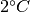. Icing causes a reduction of the efficiency.

Parameters: temp_high (list or pandas.Series of numerical values) – Temperature of the high temperature reservoir in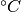temp_low (list or pandas.Series of numerical values) – Temperature of the low temperature reservoir inquality_grade (numerical value) – Factor that scales down the efficiency of the real heat pump (or chiller) process from the ideal process (Carnot efficiency), where a factor of 1 means teh real process is equal to the ideal one. factor_icing (numerical value) – Sets the relative COP drop caused by icing, where 1 stands for no efficiency-drop. mode (string) – Two possible modes: “heat_pump” or “chiller” (default ‘None’) t_threshold – Temperature inbelow which icing at heat exchanger occurs (default 2) cops – List of Coefficients of Performance (COPs) list of numerical values
oemof.thermal.compression_heatpumps_and_chillers.calc_max_Q_dot_chill(nominal_conditions, cops)[source]

Calculates the maximal cooling capacity (relative value) of a chiller.

Note

This function assumes the cooling capacity of a chiller can exceed the rated nominal capacity (e.g., from the technical specification sheet). That means: The value of max_Q_chill can be greater than 1. Make sure your actual chiller is capable of doing so. If not, use 1 for the maximal cooling capacity.

Parameters: nominal_conditions (dict) – Dictionary describing one operating point (e.g., operation under STC) of the chiller by its cooling capacity, its electricity consumption and its COP (‘nominal_Q_chill’, ‘nominal_el_consumption’ and ‘nominal_cop’) cops (list of numerical values) – Actual COP max_Q_chill – Maximal cooling capacity (relative value). Value is equal or greater than 0 and can be greater than 1. list of numerical values
oemof.thermal.compression_heatpumps_and_chillers.calc_max_Q_dot_heat(nominal_conditions, cops)[source]

Calculates the maximal heating capacity (relative value) of a heat pump.

This function assumes the heating capacity of a heat pump can exceed the rated nominal capacity (e.g., from the technical specification sheet). That means: The value of max_Q_hot can be greater than 1. Make sure your actual heat pump is capable of doing so. If not, use 1 for the maximal heating capacity.

# solar_thermal_collector module¶

This module is designed to hold functions for calculating a solar thermal collector.

This file is part of project oemof (github.com/oemof/oemof-thermal). It’s copyrighted by the contributors recorded in the version control history of the file, available from its original location: oemof-thermal/src/oemof/thermal/solar_thermal_collector.py

oemof.thermal.solar_thermal_collector.calc_eta_c_flate_plate(eta_0, a_1, a_2, temp_collector_inlet, delta_temp_n, temp_amb, collector_irradiance)[source]

Calculates collectors efficiency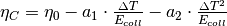with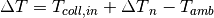Parameters: eta_0 (numeric) – Optical efficiency of the collector. a_1 (numeric) – Thermal loss parameter 1. a_2 (numeric) – Thermal loss parameter 2. temp_collector_inlet (numeric, in °C) – Collectors inlet temperature. delta_temp_n (numeric) – Temperature difference between collector inlet and mean temperature. temp_amb (series of numeric, in °C) – Ambient temperature. collector_irradiance (series of numeric) – Irradiance on collector after all losses. eta_c – collectors efficiency series of numeric
oemof.thermal.solar_thermal_collector.flat_plate_precalc(lat, long, collector_tilt, collector_azimuth, eta_0, a_1, a_2, temp_collector_inlet, delta_temp_n, irradiance_global, irradiance_diffuse, temp_amb)[source]

Calculates collectors heat, efficiency and irradiance of a flat plate collector.Parameters: lat (numeric) – Latitude of the location. long (numeric) – Longitude of the location. collector_tilt (numeric) – The tilt of the collector. collector_azimuth (numeric) – The azimuth of the collector. Azimuth according to pvlib in decimal degrees East of North. eta_0 (numeric) – Optical efficiency of the collector. a_2 (a_1,) – Thermal loss parameters. temp_collector_inlet (numeric or series with length of periods) – Collectors inlet temperature. delta_temp_n (numeric) – Temperature difference between collector inlet and mean temperature. irradiance_global (time indexed series) – Global horizontal irradiance. irradiance_diffuse (time indexed series) – Diffuse irradiance. temp_amb (time indexed series) – Ambient temperature. data – DataFrame containing the followiing columns: col_ira: The irradiance on the tilted collector. eta_c: The efficiency of the collector. collector_heat: The heat power output of the collector. pandas.DataFrame

# stratified_thermal_storage module¶

This module is designed to hold functions for calculating stratified thermal storages.

This file is part of project oemof (github.com/oemof/oemof-thermal). It’s copyrighted by the contributors recorded in the version control history of the file, available from its original location: oemof-thermal/src/oemof/thermal/stratified_thermal_storage.py

oemof.thermal.stratified_thermal_storage.calculate_capacities(volume, temp_h, temp_c, heat_capacity=4195.52, density=971.803)[source]

Calculates the nominal storage capacity, minimum and maximum storage level of a stratified thermal storage.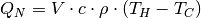Parameters: volume (numeric) – Volume of the storage [m3] temp_h (numeric) – Temperature of hot storage medium [deg C] temp_c (numeric) – Temperature of cold storage medium [deg C] heat_capacity (numeric) – Average specific heat capacity of storage medium [J/(kg*K)] Default values calculated with CoolProp for a temperature of 80 °C as a simplifying assumption density (numeric) – Average density of storage medium [kg/m3] Default values calculated with CoolProp for a temperature of 80 °C as a simplifying assumption nominal_storage_capacity – Maximum amount of stored thermal energy [MWh] numeric
oemof.thermal.stratified_thermal_storage.calculate_losses(u_value, diameter, temp_h, temp_c, temp_env, time_increment=1, heat_capacity=4195.52, density=971.803)[source]

Calculates loss rate and fixed losses for a stratified thermal storage.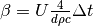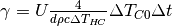Parameters: u_value (numeric) – Thermal transmittance of storage envelope [W/(m2*K)] diameter (numeric) – Diameter of the storage [m] temp_h (numeric) – Temperature of hot storage medium [deg C] temp_c (numeric) – Temperature of cold storage medium [deg C] temp_env (numeric) – Temperature outside of the storage [deg C] time_increment (numeric) – Time increment of the oemof.solph.EnergySystem [h] heat_capacity (numeric) – Average specific heat capacity of storage medium [J/(kg*K)] Default values calculated with CoolProp for a temperature of 80 °C as a simplifying assumption density (numeric) – Average density of storage medium [kg/m3] Default values calculated with CoolProp for a temperature of 80 °C as a simplifying assumption loss_rate (numeric (sequence or scalar)) – The relative loss of the storage capacity between two consecutive timesteps [-] fixed_losses_relative (numeric (sequence or scalar)) – Losses independent of state of charge between two consecutive timesteps relative to nominal storage capacity [-] fixed_losses_absolute (numeric (sequence or scalar)) – Losses independent of state of charge and independent of nominal storage capacity between two consecutive timesteps [MWh]
oemof.thermal.stratified_thermal_storage.calculate_storage_dimensions(height, diameter)[source]

Calculates volume and total surface of a hot water storage.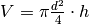Parameters: height (numeric) – Height of the storage [m] diameter (numeric) – Diameter of the storage [m] volume (numeric) – Volume of storage surface (numeric) – Total surface of storage [m2]
oemof.thermal.stratified_thermal_storage.calculate_storage_u_value(s_iso, lamb_iso, alpha_inside, alpha_outside)[source]

Calculates the thermal transmittance (U-value) of a thermal storage.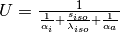Parameters: s_iso (numeric) – Thickness of isolation layer [mm] lamb_iso (numeric) – Thermal conductivity of isolation layer [W/(m*K)] alpha_inside (numeric) – Heat transfer coefficient at the inner surface of the storage [W/(m2*K)] alpha_outside (numeric) – Heat transfer coefficient at the outer surface of the storage [W/(m2*K)] u_value – Thermal transmittance (U-value) [W/(m2*K)] numeric

Facade’s are classes providing a simplified view on more complex classes. More specifically, the Facade s in this module inherit from oemof.solph’s generic classes to serve as more concrete and energy specific interface.

The concept of the facades has been derived from oemof.tabular. The idea is to be able to instantiate a Facade using only keyword arguments. Under the hood the Facade then uses these arguments to construct an oemof.solph component and sets it up to be easily used in an EnergySystem. Usually, a subset of the attributes of the parent class remains while another part can be addressed by more specific or simpler attributes.

Note The mathematical notation is as follows:

• Optimization variables (endogenous) are denoted by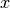• Optimization parameters (exogenous) are denoted by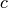• The set of timesteps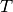describes all timesteps of the optimization problem

class oemof.thermal.facades.Facade(*args, **kwargs)[source]

Bases: oemof.network.network.Node

Parameters: _facade_requires_ (list of str) – A list of required attributes. The constructor checks whether these are present as keyword arguments or whether they are already present on self (which means they have been set by constructors of subclasses) and raises an error if he doesn’t find them.
update()[source]
class oemof.thermal.facades.ParabolicTroughCollector(*args, **kwargs)[source]

Bases: oemof.solph.network.transformer.Transformer, oemof.thermal.facades.Facade

Parabolic trough collector unit

Parameters: heat_bus (oemof.solph.Bus) – An oemof bus instance in which absorbs the collectors heat. electrical_bus (oemof.solph.Bus) – An oemof bus instance which provides electrical energy to the collector. electrical_consumption (numeric) – Specifies how much electrical energy is used per provided thermal energy. additional_losses (numeric) – Specifies how much thermal energy is lost in peripheral parts like pipes and pumps. aperture_area (numeric) – Specify the ares or size of the collector.

See the API of csp_precalc in oemof.thermal.concentrating_solar_power for the other parameters.

Examples

>>> from oemof import solph
>>> bth = solph.Bus(label='thermal_bus')
>>> bel = solph.Bus(label='electrical_bus')
>>> collector = ParabolicTroughCollector(
...     label='solar_collector',
...     heat_bus=bth,
...     electrical_bus=bel,
...     electrical_consumption=0.05,
...     aperture_area=1000,
...     loss_method='Janotte',
...     latitude=23.614328,
...     longitude=58.545284,
...     collector_tilt=10,
...     collector_azimuth=180,
...     x=0.9,
...     a_1=-0.00159,
...     a_2=0.0000977,
...     eta_0=0.816,
...     c_1=0.0622,
...     c_2=0.00023,
...     temp_collector_inlet=435,
...     temp_collector_outlet=500,
...     temp_amb=input_data['t_amb'],
... )

build_solph_components()[source]
class oemof.thermal.facades.SolarThermalCollector(*args, **kwargs)[source]

Bases: oemof.solph.network.transformer.Transformer, oemof.thermal.facades.Facade

Solar thermal collector unit

heat_out_bus: oemof.solph.Bus
An oemof bus instance which absorbs the collectors heat.
electrical_in_bus: oemof.solph.Bus
An oemof bus instance which provides electrical energy to the collector.
electrical_consumption: numeric
Specifies how much electrical energy is used per provided thermal energy.
peripheral_losses: numeric
Specifies how much thermal energy is lost in peripheral parts like pipes and pumps as percentage of provided thermal energy.
aperture_area: numeric
Specifies the size of the collector as surface area.

See the API of flat_plate_precalc in oemof.thermal.solar_thermal_collector for the other parameters.

>>> from oemof import solph
>>> bth = solph.Bus(label='thermal')
>>> bel = solph.Bus(label='electricity')
>>> collector = SolarThermalCollector(
...     label='solar_collector',
...     heat_out_bus=bth,
...     electricity_in_bus=bel,
...     electrical_consumption=0.02,
...     peripheral_losses=0.05,
...     aperture_area=1000,
...     latitude=52.2443,
...     longitude=10.5594,
...     collector_tilt=10,
...     collector_azimuth=20,
...     eta_0=0.73,
...     a_1=1.7,
...     a_2=0.016,
...     temp_collector_inlet=20,
...     delta_temp_n=10,
...     temp_amb=input_data['temp_amb'],
)

build_solph_components()[source]
class oemof.thermal.facades.StratifiedThermalStorage(label=None, inputs=None, outputs=None, nominal_storage_capacity=None, initial_storage_level=None, investment=None, invest_relation_input_output=None, invest_relation_input_capacity=None, invest_relation_output_capacity=None, min_storage_level=0.0, max_storage_level=1.0, balanced=True, loss_rate=0, fixed_losses_relative=0, fixed_losses_absolute=0, inflow_conversion_factor=1, outflow_conversion_factor=1, custom_attributes=None, **kwargs)[source]

Bases: oemof.solph.components.generic_storage.GenericStorage, oemof.thermal.facades.Facade

Stratified thermal storage unit.

Parameters: bus (oemof.solph.Bus) – An oemof bus instance where the storage unit is connected to. diameter (numeric) – Diameter of the storage [m] height (numeric) – Height of the storage [m] temp_h (numeric) – Temperature of the hot (upper) part of the water body. temp_c (numeric) – Temperature of the cold (upper) part of the water body. temp_env (numeric) – Temperature of the environment. heat_capacity (numeric) – Assumed constant for heat capacity of the water. density (numeric) – Assumed constant for density of the water. u_value (numeric) – Thermal transmittance [W/(m2*K)] capacity (numeric) – Maximum production capacity [MW] efficiency (numeric) – Efficiency of charging and discharging process: Default: 1 marginal_cost (numeric) – Marginal cost for one unit of output. expandable (boolean) – True, if capacity can be expanded within optimization. Default: False. storage_capacity_cost (numeric) – Investment costs for the storage unit [Eur/MWh]. capacity_cost (numeric) – Investment costs for charging/dischargin [Eur/MW] storage_capacity_potential (numeric) – Potential of the investment for storage capacity [MWh] capacity_potential (numeric) – Potential of the investment for capacity [MW] input_parameters (dict (optional)) – Set parameters on the input edge of the storage (see oemof.solph for more information on possible parameters) output_parameters (dict (optional)) – Set parameters on the output edge of the storage (see oemof.solph for more information on possible parameters)

The attribute nominal_storage_capacity of the base class GenericStorage should not be passed because it is determined internally from height and parameter.

Examples

>>> from oemof import solph
>>> heat_bus = solph.Bus(label='heat_bus')
>>> thermal_storage = StratifiedThermalStorage(
...     label='thermal_storage',
...     bus=heat_bus,
...     diameter=10,
...     height=10,
...     temp_h=95,
...     temp_c=60,
...     temp_env=10,
...     u_value=0.3,
...     initial_storage_level=0.5,
...     min_storage_level=0.05,
...     max_storage_level=0.95
...     capacity=1)

build_solph_components()[source]
oemof.thermal.facades.add_subnodes(n, **kwargs)[source]